ECMAScript Regular Expressions Tutorial with Examples

View more Tutorials: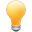Follow us on our fanpages to receive notifications every time there are new articles.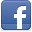Facebook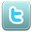Twitter

1- Regular Expression

A regular expression defines a search pattern for strings. Regular expressions can be used to search, edit and manipulate text. The pattern defined by the regular expression may match one or several times or not at all for a given string.

The abbreviation for regular expression is regex.
Regular expressions are supported by most programming languages, e.g., Java, C#, C/C++, etc. Unfortunately each language supports regular expressions slightly different.
You may be interested:

2- Rule writing regular expressions

 No Regular Expression Description 1 . Matches any character 2 ^regex Finds regex that must match at the beginning of the line. 3 regex\$ Finds regex that must match at the end of the line. 4 [abc] Set definition, can match the letter a or b or c. 5 [abc][vz] Set definition, can match a or b or c followed by either v or z. 6 [^abc] When a caret appears as the first character inside square brackets, it negates the pattern. This can match any character except a or b or c. 7 [a-d1-7] Ranges: matches a letter between a and d and figures from 1 to 7. 8 X|Z Finds X or Z. 9 XZ Finds X directly followed by Z. 10 \$ Checks if a line end follows. 11 \d Any digit, short for [0-9] 12 \D A non-digit, short for [^0-9] 13 \s A whitespace character, short for [ \t\n\x0b\r\f] 14 \S A non-whitespace character, short for [^\s] 15 \w A word character, short for [a-zA-Z_0-9] 16 \W A non-word character [^\w] 17 \S+ Several non-whitespace characters 18 \b Matches a word boundary where a word character is [a-zA-Z0-9_]. 19 * Occurs zero or more times, is short for {0,} 20 + Occurs one or more times, is short for {1,} 21 ? Occurs no or one times, ? is short for {0,1}. 22 {X} Occurs X number of times, {} describes the order of the preceding liberal 23 {X,Y} Occurs between X and Y times, 24 *? ? after a quantifier makes it a reluctant quantifier. It tries to find the smallest match.

3- Special characters in ECMAScript Regex

Special characters in ECMAScript Regex:

\.[{(*+?^\$|

The characters listed above are special characters. In ECMAScript Regex you want it understood that character in the normal way you should add a \ in front.

Example dot character . ECMAScript Regex is interpreted as any character, if you want it interpreted as a dot character normally required mark \ ahead.

// Regex pattern describe any character.
let regex1 = /./ ;

// Regex pattern describe a dot character.
let regex2 = /\./ ;

4- Create a RegExp

There are 2 ways to create a RegExp object.

// Create via constructor of RegExp.
var pattern = new RegExp(pattern, attributes);

//
var pattern = /pattern/attributes;
Attribute: m (Multiple)
If a string contains new line character ( \n ), you can use this character to parse the original string into multiple substrings. A regular expression with the m (Multiple) attribute tells the program to parse the initial string into multiple substrings as described above, and then perform the current duty with each of them.
Attribute: i (Ignore Case)
The i (Ignore Case) attribute tells the program that it is not interested in uppercase or lowercase letters while comparing.
Attribute: g (Global)
The g attribute, which stands for Global.
Note: You will understand more about the g (Global) attribute in the examples.
Attribute: u (Unicode)
This attribute says to the program "treat the target String as an Unicode text. An Unicode character can be Encoded by 2, 3 or 4 bytes.
Attribute: y (Sticky)

5- Basic examples

aRegex.test(aString) is the most basic method helping you test a aString string by the rules.: If you find that a substring of the aString string matches the aRegex regular expression, it will return true, on the contrary, it will return false.The basic following examples help you understand the rules for setting up a regular expression more.
Example 1:
regex-test-example1.js

// A Regular Expression with Rule:
// ABC, followed by any character appears one or more times.
// Rules: ., +
let aRegex = /ABC.+/

let str1 = "AB DE";

// Exists a substring of string str1 matching aRegex?
let result1 = aRegex.test(str1);

console.log(result1); // false

let str2 = "123 ABC";
//
let result2 = aRegex.test(str2);

console.log(result2); // false

let str3 = "123 ABCdef";
//
let result3 = aRegex.test(str3);

console.log(result3); // true
Example 2:
regex-test-example2.js

// A Regular Expression with Rule:
// ABC, followed by x or y or z
// Next, followed by any character appears one or more times.
// Rule: [abc], . , +
let aRegex = /ABC[xyz].+/

let str1 = "123ABCx";
// Exists a substring of string str1 matching aRegex?
let result1 = aRegex.test(str1);

console.log(result1); // false

let str2 = "123ABCzMNP";
//
let result2 = aRegex.test(str2);

console.log(result2); // true
Example 3:
regex-test-example3.js

// A Regular Expression with Rule:
// ABC or JERRY
// Next, followed by any character appears one or more times.
// Next, followed by CAT
// Rule: (X|Z), . , +
let aRegex = /(TOM|JERRY).+CAT/

let str1 = "I saw TOM, TOM caught JERRY";
// Exists a substring of string str1 matching aRegex?
let result1 = aRegex.test(str1);

console.log(result1); // false

let str2 = "I saw TOM, TOM is a CAT";
//
let result2 = aRegex.test(str2);

console.log(result2); // true

let str3 = "JERRY is a mouse, It is afraid of the CAT";
//
let result3 = aRegex.test(str3);

console.log(result3); // true
Example 4:
regex-test-example4.js

// A Regular Expression with Rule:
// Next, followed by any character appears one or more times.
// Next, followed by CAT, and ends.
// Rule: ^, (X|Z), . , +, \$
let aRegex = /^(TOM|JERRY).+CAT\$/

let str1 = "I saw TOM, TOM caught JERRY";
// Exists a substring of string str1 matching aRegex?
let result1 = aRegex.test(str1);

console.log(result1); // false

let str2 = "I saw TOM, TOM is a CAT";
//
let result2 = aRegex.test(str2);

console.log(result2); // false

let str3 = "JERRY is a mouse, It is afraid of the CAT";
//
let result3 = aRegex.test(str3);

console.log(result3); // true
Example 5:
regex-test-example5.js

// A Regular Expression with Rule:
// Start with a digit, appears 5 to 7 times, and Ends.
// Rule: ^, \d, {X,Y}, \$
let aRegex = /^\d{5,7}\$/

let str1 = "The Result is: 12345";
// Exists a substring of string str1 matching aRegex?
let result1 = aRegex.test(str1);

console.log(result1); // false

let str2 = "A12345";
//
let result2 = aRegex.test(str2);

console.log(result2); // false

let str3 = "912345";
//
let result3 = aRegex.test(str3);

console.log(result3); // true
Example 6 (Attribute: m)
Example of a regular expression with the m (Multiple Line) parameter.
regex-test-attr-m-example.js

// A Regular Expression with Rule:
// Start with a digit, appears 5 to 7 times, and Ends.
// Rule: ^, \d, {X,Y}, \$
let aRegex = /^\d{5,7}\$/m

let str1 = "The Result is: 12345";
//
let result1 = aRegex.test(str1);

console.log(result1); // false

// This string is splitted into two substrings
// 'The Result is:' & '12345'
let str2 = "The Result is:\n12345";
//
let result2 = aRegex.test(str2);

console.log(result2); // true
Example 7 (Attribute: i)
Example of a regular expression with the i (Ignore Case) parameter. The algorithm will not care about upper and lower case differences while matching.
regex-test-attr-i-example.js

// A Regular Expression with Rule:
// ABC or JERRY
// Next, followed by any character appears one or more times.
// Next, followed by CAT
// Rule: (X|Z), . , +
let aRegex = /(TOM|JERRY).+CAT/i

let str1 = "I saw TOM, TOM is a cat";
// Exists a substring of string str1 matching aRegex?
let result1 = aRegex.test(str1);

console.log(result1); // false

let str2 = "I saw TOM, TOM is a CAT";
//
let result2 = aRegex.test(str2);

console.log(result2); // true

6- Methods related to RegExp

RegExp.exec(string)
This method looks for a substring that matches a regular expression. If it finds, it returns an array of shapes such as [substring, index: idx, input: currentString], on the contrary, it returns null. The location of finding the suitable substring which is index: idx will be used as the starting point for the next search. If the regular expression has the g (Global) parameter, on the contrary, the search starting point is 0.
For example, Use the RegExp.exec(string) method, of which the regular expression has no the g (Global) parameter:
regex-exec-example1.js

// A Regular Expression with Rule:
// ABC, followed by any digit appears one or more times.
// Rules: ., \d, +
let aRegex = /ABC\d+/

let str1 = "ABC 123";

// Find a substring of string str1 matching aRegex?
let result1 = aRegex.exec(str1);

console.log(result1); // null

//
let str2 = "123 ABC123 ABC45 x";
let result2 = aRegex.exec(str2);

console.log(result2); // [ 'ABC123', index: 4, input: '123 ABC123 ABC45 x' ]

console.log(result2); // ABC123
console.log(result2["index"]); // 4
console.log(result2["input"]); // 123 ABC123 ABC45 x

null
[ 'ABC123', index: 4, input: '123 ABC123 ABC45 x']
ABC123
4
123 ABC123 ABC45 x
For example, using the RegExp.exec(string) method, the regular expression has the g (Global) parameter:
regex-exec-attr-g-example.js

// A Regular Expression with Rule:
// Any digit appears one or more times.
// Rules:  \d, +
let aRegex = /\d+/g

let str1 = "ABC 123 X22 345";

let array1;

do {
array1 = aRegex.exec(str1);
console.log(array1);
} while( array1 != null);
String.split()
This method splits the current string into substrings, and returns an array of substrings.

var substrings = str.split([separator[, limit]])
Parameters:
• separator: A regular expression, or a String used to separate current string.
• limit: Limit on quantity of strings returned.
string-split-example.js

// A Regular Expression with Rule:
// Whitespace character, appear one or more times
// Rules:  \s, +
let aRegex = /\s+/

let str1 = "Banana \t\t Apple Lemon";

let result1 = str1.split(aRegex);

console.log(result1); // [ 'Banana', 'Apple', 'Lemon' ]
String.search()
This method returns index where substring of current string suitable for regular expression is found. If no finding, it will return -1.

var index = str.search(searchValue)
Parameters:
• searchValue: Regular expression or a String for searching.
string-replace-example.js

// A Regular Expression with Rule:
// TOM or JERRY
// Rule:  (X|Z)
let aRegex = /(TOM|JERRY)/

let str = "I saw TOM, TOM caught JERRY";

let index = str.search(aRegex);

console.log(index); // 6
String.replace(search, newSubString)
This method searches the first substring matching a regular expression to replace it with a new substring. If the regular expression has the g (Global) parameter, the method will search  all substrings that match the regular expression to replace with the new substring.

str.replace(regexp|substr, newSubStr|aFunction)

Parameters:
• regexp: A regular expression.
• substr: A substring.
• newSubStr:  replacement string .
• aFunction: A function that returns an replacement string.
For example, use the String.replace() method to replace all blanks appearing one or more times in a string by a (-) character
string-replace-example.js

// A Whitespace Characters, appears one or more times.
// Rule: \s, +
let aRegex = /\s+/g

let str = "This \t\t is a \n\t text";

str = str.replace(aRegex, '-');

console.log(str); // This-is-a-text

7- Group

A regular expression you can split into groups:

// A regular expression
let regex1 = /\w+=\d+/;

// Writing as 2 groups
let regex2 = /(\w+)=(\d+)/;

// 3 groups
let regex3 = /(\w+)(=)(\d+)/;
The group can be nested, and so need a rule indexing the group. The entire pattern is defined as the group 0. The remaining group described similar illustration below: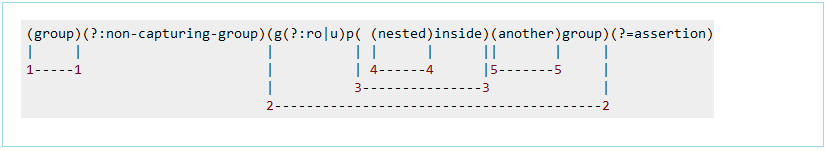indexed-group-example.js

let regex1 = /\w+=\d+/;

let regex2 = /(\w+)=(\d+)/;

let regex3 = /(\w+)(=)(\d+)/;

var STR = "abc=100";

let result1 = regex1.exec(STR);
console.log(result1);// [ 'abc=100', index: 0, input: 'abc=100' ]

let result2 = regex2.exec(STR);
console.log(result2);// [ 'abc=100', 'abc', '100', index: 0, input: 'abc=100' ]

let result3 = regex3.exec(STR);
console.log(result3);// [ 'abc=100', 'abc', '=', '100', index: 0, input: 'abc=100' ]
Output:

[ 'abc=100', index: 0, input: 'abc=100' ]
[ 'abc=100', 'abc', '100', index: 0, input: 'abc=100' ]
[ 'abc=100', 'abc', '=', '100', index: 0, input: 'abc=100' ]

Note: Use (?:pattern) to inform ECMAScript does not see this as a group (None-capturing group)

none-capturing-group-example.js

// Date Format: yyyy-MM-dd
let dateRegex = /([0-9]{4})-(?:[0-9]{2})-([0-9]{2})/;

var STR = "2001-11-24";

let result  = dateRegex.exec(STR);
console.log(result);// [ '2001-11-24', '2001', '24', index: 0, input: '2001-11-24' ]

console.log("Date: " + result); // Date: 2001-11-24
console.log("Year: " + result); // Year: 2001
console.log("Day: " + result);  // Day: 24
Output:

[ '2001-11-24', '2001', '24', index: 0, input: '2001-11-24' ]
Date: 2001-11-24
Year: 2001
Day: 24
Following is a more complex example. This example will show you the benefits of using a group in regular expressions.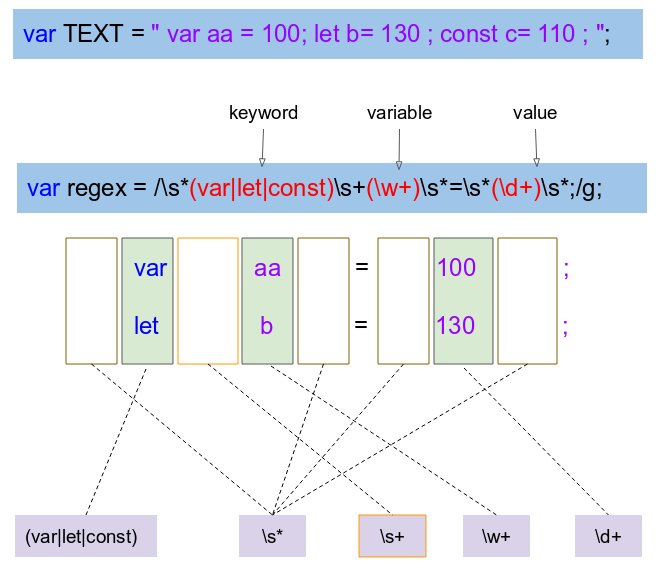(var|let|const) var or let or const. \s* The whitespace character appears 0 or more times. \s+ The whitespace character appears 1 or more times. \w+ Letter character appears 1 or more times. \d+ Number characters appear one or more times.
indexed-group-example2.js

var TEXT = " var aa = 100; let b= 130 ; const c= 110 ; ";

var regex = /\s*(var|let|const)\s+(\w+)\s*=\s*(\d+)\s*;/g;

var result;

while (result = regex.exec(TEXT) ) {

console.log("substring: " + result);
console.log("keyword: " + result);
console.log("variable: " + result);
console.log("value: " + result);
console.log("------------------------------");
}

Output:

substring: var aa = 100;
keyword: var
variable: aa
value: 100
------------------------------
substring: let b= 130 ;
keyword: let
variable: b
value: 130
------------------------------
substring: const c= 110 ;
keyword: const
variable: c
value: 110
------------------------------

View more Tutorials:

Maybe you are interested

These are online courses outside the o7planning website that we introduced, which may include free or discounted courses.

•The Complete JavaScript Bootcamp
•Getting really good at JavaScript and TypeScript
•Essentials of JavaScript Practice Coding Exercises Tips
•Learn JavaScript From Scratch:Become Top Rated Web Developer
•JavaScript For Beginners - Learn JavaScript From Scratch
•Javascript for Beginners
•Learning ECMAScript 6: Moving to the New JavaScript
•JavaScript Intro to learning JavaScript web programming
•JavaScript Dynamic Quiz Application from Scratch JSON AJAX
•Byte-Sized-Chunks: Dynamic Prototypes in Javascript
•Learn ECMAScript 2015 - ES6 Best Course
•Getting started with javascript and its core concepts
•Quick JavaScript Core learning Course JavaScript Essentials
•HTML CSS JavaScript: Most popular ways to code HTML CSS JS
•*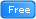* Master ECMAScript 2015 (ES6)
•Learn JavaScript Fundamentals
•** Start 3D GIS Web Development in JavaScript
•Learning JavaScript Programming Tutorial. A Definitive Guide
•2D Game Development With HTML5 Canvas, JS - Tic Tac Toe Game
•JavaScript in 55 Minutes
•JavaScript for Beginning Web Developers
•The Web Development Course: HTML5, CSS3, JavaScript
•The complete beginner JavaScript ES5, ES6 and JQuery Course
•JavaScript in Action JavaScript Projects
•** Introductory To JavaScript - Learn The Basics of JavaScript